Latest Banking jobs   »   Reasoning Ability Quiz For IBPS RRB...

# Reasoning Ability Quiz For IBPS RRB PO, Clerk Prelims 2021- 9th August

Directions (1-5): Study the information carefully and answer the questions given below.
There are eight boxes J, K, L, M, N, O, P and Q are placed one above another. Two boxes are placed between Box K and Box N. Box Q is placed immediately below Box N. One box is placed between Box P and Box L. Box M is placed one of the above place of N and one of the below place of Box O but not immediate above or below. Box J and Box O is not placed above Box P.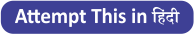Q1. Which of the following box is placed just below box L?
(a) N
(b) Q
(c) M
(d) O
(e) None of these

Q2. Which of the following box is placed just above box N?
(a) Q
(b) L
(c) M
(d) J
(e) None of these

Q3. How many boxes are placed between box O and box N?
(a) Three
(b) Two
(c) One
(d) Four
(e) None

Q4. What is the position of box M?
(a) Third from the top
(b) Second from the top
(c) Third from the bottom
(d) Second from the bottom
(e) None of these

Q5. Four of the following five are alike in certain way based from a group, find the one that does not belong to that group?
(a) P-L
(b) O-K
(c) N-M
(d) L-N
(e) Q-J

Directions (6-10): Study the following information carefully and answer the questions given below:
In a certain code language
‘where house plan army’ is written as ‘nl am hr ou’,
‘ball house birth data’ is written as ‘tm rb lb hr’,
‘army plan register already’ is written as ‘ed nl am tr’,
‘fade plan data ball’ is written as ‘tm lb nl dm’.

Q6. What is the code for ‘Fade’?
(a) dm
(b) nl
(c) lb
(d) tm
(e) None of these

Q7. Which of the following may be the code for ‘already house plan’?
(a) ed hr rb
(b) tr nl hr
(c) rb hr tr
(d) ed nl hr
(e) Either (b) or (d)

Q8. What is the code for ‘Register’?
(a) tr
(b) am
(c) nl
(d) ed
(e) Can’t be determined

Q9. What does ‘rb’ stand for?
(a) House
(b) Ball
(c) Birth
(d) Data
(e) None of these

Q10. What is the code for ‘Plan’?
(a) am
(b) hr
(c) lb
(d) nl
(e) Can’t be determined

Direction (11-15): Study the following alphanumeric series carefully and answer the questions given below:
S * 7 B \$ * G 2 8 U 1 & A V # F 3 5 H @ 5 Y 5 M 3 2 D 8 % N 2 4

Q11. How many such numbers are there which is immediately preceded by alphabets and immediately followed by symbols?
(a) One
(b) Two
(c) Three
(d) Four
(e) None

Q12. Which of the following element is 19th from right end of the series?
(a) #
(b) F
(c) 3
(d) V
(e) None of these

Q13. How many such symbols are there which is immediately preceded by numbers and immediately followed by vowels?
(a) Two
(b) None
(c) One
(d) Four
(e) Three

Q14.How many such alphabets are there which is immediately preceded and followed by same number?
(a) Three
(b) Two
(c) One
(d) Four
(e) None

Q15. If all numbers are eliminated from the series then, which of the following element is 11th from right end of the series?
(a) V
(b) A
(c) &
(d) #
(e) None of these

Solutions

Solutions (1-5):
Sol.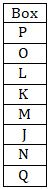S1. Ans(e)
S2. Ans(d)
S3. Ans(d)
S4. Ans(e)
S5. Ans(d)

Solution (6-10):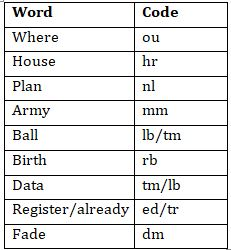S6. Ans.(a)
S7. Ans.(e)
S8. Ans.(e)
S9. Ans.(c)
S10. Ans.(d)

S11. Ans(b)
Sol. U1&, D8%

S12. Ans(d)

S13. Ans(c)
Sol. 1&A

S14. Ans(c)
Sol. 5 Y 5

S15.Ans(b)#### Congratulations!

Incorrect details? Fill the form again here

•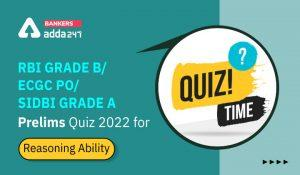Reasoning Ability Quiz For RBI Grade B/ ...
•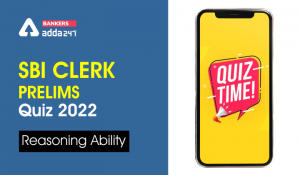Reasoning Ability Quiz For SBI Clerk Pre...
•Reasoning Ability Quiz For RBI Grade B/ ...
•Reasoning Ability Quiz For SBI Clerk Pre...
•Reasoning Ability Quiz For SBI Clerk Pre...
•Reasoning Ability Quiz For SBI Clerk Pre...